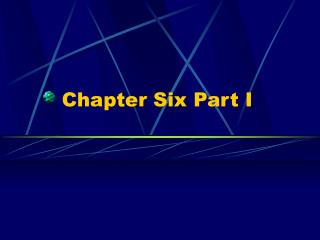Download PresentationChapter Six Part I

# Chapter Six Part I

Download Presentation## Chapter Six Part I

- - - - - - - - - - - - - - - - - - - - - - - - - - - E N D - - - - - - - - - - - - - - - - - - - - - - - - - - -
##### Presentation Transcript

1. Chapter Six Part I

2. Questions of the Day • What an atom? How are they made? • What is spectroscopy? How is it used in Astronomy? • How are atoms and spectroscopy related? Why is this important to astronomy?

3. Atoms • They emit and absorb energy • Elements are unique atomic structures • Element A + Element B = Compound • Unit of a compound: Molecule

4. An Atom

5. Atomic Structure and Spectra • Electrons • J. J. Tomson (1897) • Cathode ray tubes • Negative versus Positive • Created electric field around the ray tube • Negative charged particles flowed away from positive terminal.

6. Atomic Structure (cont.) • Electrons (cont.) • Robert Millikan (1906) • Charged oil drops were deflected using charged plates • Tomson had given limits to mass of electrons, but Millikan found the q/m • Masselectron = 9.11 x 10-11 kg • Tomson suggest raisin in plum pudding model • Another structure was suggested by presence of alpha, beta, and gamma particles

7. Atomic Structure (cont.) • Nucleus • Ernst Rutherford (1907) • Focused a beam of alpha particles through a thin sheet of Au (Gold) then detected behind by zinc sulfide that sparked when alpha hit it. • Most particles went through the sheet of Au, but some were back scattered by a small object, assumed to be a nucleus • Regions assumed to be 10-13 to 10-8 cm

8. Atomic Structure (cont.) • Nucleus (cont.) • Rutherford (1911) • Used alpha to break up the nucleus of a N (nitrogen) atom and found 7 discrete unit of charge like the electrons, but positively charged • Called the particles protons • Lead to atomic number for each element • Maxwell put the proton and electron together with the electron orbiting the proton

9. Bohr Model of the Atom • Bohr’s theory • Allowed orbits have angular momentum conserved and angular force corrected • mvr = nh/2 • kq1q2/r2 = mv2/r • Solving the equations simultaneously we get the Bohr radius of 0.529 X 10-10 m

10. Bohr Model (cont.) • Stable orbits do not give off radiation, but the 21 cm line is an exception to this rule • Quantum jumps: used the H (hydrogen) atom • En = EI /n2 • hf = EH – EI • Bohr model worked wonderfully for H, but did not work for higher elements so we needed a new science, Quantum Mechanics

11. Fig.06.07

12. Fig.06.08

13. Quantum Mechanics http://ahs.wcs.k12.va.us/Chem%20Web%20Final_files/image012.jpg

14. Spectra and Elements http://jersey.uoregon.edu/elements/Elements.html

15. Fig.06.01

16. The Speed of Light

17. Electromagnetic Spectrum http://www.lbl.gov/MicroWorlds/ALSTool/EMSpec/

19. Gustav Kirchoff • 1824 –1887 • Student of Gauss • Taught at Berlin, Brausla, and Hiedelberg • Vorleschugen uber Matematische Physik • Created laws for electrical circuits, spectroscopy, and topology in mathematics http://www.hao.ucar.edu/public/education/sp/images/kirchhoff.jpg

20. Kirchoff’s First Rule • A hot and opaque solid, liquid, or highly compressed gas emits a continuous spectrum. • Example: Light Bulb filament

21. Kirchoff’s Second Rule • A hot, transparent gas produces a spectrum of bright lines (emission lines). The number of these lines depend on which elements are present in the gas. • Example: A neon sign

22. Kirchoff’s Third Rule • If a continuous spectrum (from a hot, opaque solid, liquid, or gas) passes through a transparent gas at a lower temperature, the cooler gas will cause the appearance of dark lines (absorption lines). • Example: Light from the Sun.

23. Temperature and Brightness • Wien’s Law • BlackBody.html

24. Wave Nature of Light • Diffraction • Huygens • Light bending around an opaque object • Interference • Young’s Double slit experiment

25. Diffraction http://www.stsci.edu

26. Particle Nature of Light • Photoelectric effect • Quanta • E = hf • Lenses

27. Lens http://www.stsci.edu

28. A “Wavicle”

29. The Doppler Effect

30. Homework 6 • What are the parts of the electromagnetic spectrum? What part can we see? What everyday objects emit each part of the spectrum? • What is the frequency of light that is 6.365X10-10m? (Remember that the speed of light is 3.000X108 m/s) • Page 127 CQ 1,2 P 2 • What is the energy of the particle of light with a wavelength of 6.365X10-10m? (Remember the Heisenberg uncertainty number, h, is 6.63X10-34Js)